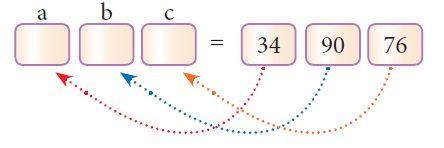#### Tuple in Python

Tuples consists of a number of values separated by comma and enclosed within parentheses. Tuple is similar to list, values in a list can be changed but not in a tuple.

The term Tuple is originated from the Latin word represents an abstraction of the sequence of numbers:
single(1), double(2), triple(3), quadruple(4), quintuple(5), sextuple(6), septuple(7), octuple(8), ..., n‑tuple, ...,

1. The elements of a list are changeable (mutable) whereas the elements of a tuple are unchangeable (immutable), this is the key difference between tuples and list.

2. The elements of a list are enclosed within square brackets. But, the elements of a tuple are enclosed by paranthesis.

3. Iterating tuples is faster than list.

Creating Tuples

Creating tuples is similar to list. In a list, elements are defined within square brackets, whereas in tuples, they may be enclosed by parenthesis. The elements of a tuple can be even defined without parenthesis. Whether the elements defined within parenthesis or without parenthesis, there is no differente in it's function.

Syntax:

`# Empty tupleTuple_Name = ( )# Tuple with n number elementsTuple_Name = (E1, E2, E2 ……. En)# Elements of a tuple without parenthesisTuple_Name = E1, E2, E3 ….. En`

Example

`>>> MyTup1 = (23, 56, 89, 'A', 'E', 'I', "Tamil")>>> print(MyTup1)(23, 56, 89, 'A', 'E', 'I', 'Tamil')>>> MyTup2 = 23, 56, 89, 'A', 'E', 'I', "Tamil">>> print (MyTup2)(23, 56, 89, 'A', 'E', 'I', 'Tamil')`

(i) Creating tuples using tuple( ) function

The tuple( ) function is used to create Tuples from a list. When you create a tuple, from a list, the elements should be enclosed within square brackets.

Syntax:

`Tuple_Name = tuple( [list elements] )`

Example

`>>> MyTup3 = tuple( [23, 45, 90] )>>> print(MyTup3)(23, 45, 90)>>> type (MyTup3)<class ‘tuple’>`

(ii) Creating Single element tuple

While creating a tuple with a single element, add a comma at the end of the element. In the absence of a comma, Python will consider the element as an ordinary data type; not a tuple. Creating a Tuple with one element is called “Singleton” tuple.

Example

`>>> MyTup4 = (10)>>> type(MyTup4)<class 'int'>>>> MyTup5 = (10,)>>> type(MyTup5)<class 'tuple'>`

Accessing values in a Tuple

Like list, each element of tuple has an index number starting from zero. The elements of a tuple can be easily accessed by using index number.

Example

`>>> Tup1 = (12, 78, 91, “Tamil”, “Telugu”, 3.14, 69.48)# to access all the elements of a tuple>>> print(Tup1)(12, 78, 91, 'Tamil', 'Telugu', 3.14, 69.48)#accessing selected elements using indices>>> print(Tup1[2:5])(91, 'Tamil', 'Telugu')#accessing from the first element up to the specified index value>>> print(Tup1[:5])(12, 78, 91, 'Tamil', 'Telugu')# accessing from the specified element up to the last element.>>> print(Tup1[4:])('Telugu', 3.14, 69.48)# accessing from the first element to the last element>>> print(Tup1[:])(12, 78, 91, 'Tamil', 'Telugu', 3.14, 69.48)`

Update and Delete Tuple

As you know a tuple is immutable, the elements in a tuple cannot be changed. Instead of altering values in a tuple, joining two tuples or deleting the entire tuple is possible.

Example

`# Program to join two tuplesTup1 = (2,4,6,8,10)Tup2 = (1,3,5,7,9)Tup3 = Tup1 + Tup2print(Tup3)`

Output

(2, 4, 6, 8, 10, 1, 3, 5, 7, 9)

To delete an entire tuple, the del command can be used.

Syntax:

`del tuple_name`

Example

`Tup1 = (2,4,6,8,10)print("The elements of Tup1 is ", Tup1)del Tup1print (Tup1)`

Output:

`The elements of Tup1 is (2, 4, 6, 8, 10)Traceback (most recent call last):File "D:/Python/Tuple Examp 1.py", line 4, in <module>print (Tup1)NameError: name 'Tup1' is not defined`

Tuple Assignment

Tuple assignment is a powerful feature in Python. It allows a tuple variable on the left of the assignment operator to be assigned to the values on the right side of the assignment operator. Each value is assigned to its respective variable.Example

`>>> (a, b, c) = (34, 90, 76)>>> print(a,b,c)34 90 76# expression are evaluated before assignment>>> (x, y, z, p) = (2**2, 5/3+4, 15%2, 34>65)>>> print(x,y,z,p)4 5.666666666666667 1 False`

Note that, when you assign values to a tuple, ensure that the number of values on both sides of the assignment operator are same; otherwise, an error is generated by Python.

Returning multiple values in Tuples

A function can return only one value at a time, but Python returns more than one value from a function. Python groups multiple values and returns them together.

Example : Program to return the maximum as well as minimum values in a list

`def Min_Max(n):	a = max(n)	b = min(n)	return(a, b)Num = (12, 65, 84, 1, 18, 85, 99)(Max_Num, Min_Num) = Min_Max(Num)print("Maximum value = ", Max_Num)print("Minimum value = ", Min_Num)`

Output:

Maximum value = 99

Minimum value = 1

Nested Tuples

In Python, a tuple can be defined inside another tuple; called Nested tuple. In a nested tuple, each tuple is considered as an element. The for loop will be useful to access all the elements in a nested tuple.

Example

`Toppers = (("Vinodini", "XII-F", 98.7), ("Soundarya", "XII-H", 97.5),	("Tharani", "XII-F", 95.3), ("Saisri", "XII-G", 93.8))for i in Toppers:	print(i)`

Output:

`('Vinodini', 'XII-F', 98.7)('Soundarya', 'XII-H', 97.5)('Tharani', 'XII-F', 95.3)('Saisri', 'XII-G', 93.8)`